JEE  >  Part Test - 16 (JEE Advanced)

# Part Test - 16 (JEE Advanced)

Test Description

## 60 Questions MCQ Test JEE Main & Advanced Mock Test Series | Part Test - 16 (JEE Advanced)

Part Test - 16 (JEE Advanced) for JEE 2023 is part of JEE Main & Advanced Mock Test Series preparation. The Part Test - 16 (JEE Advanced) questions and answers have been prepared according to the JEE exam syllabus.The Part Test - 16 (JEE Advanced) MCQs are made for JEE 2023 Exam. Find important definitions, questions, notes, meanings, examples, exercises, MCQs and online tests for Part Test - 16 (JEE Advanced) below.
 1 Crore+ students have signed up on EduRev. Have you?
Part Test - 16 (JEE Advanced) - Question 1

### A person of mass 60 kg is inside a lift of mass 940 kg and presses the button one control panel. The lift starts moving upwards with an acceleration 1.0 m/s2. If g = 10 ms–2, the tension in the supporting cable is

Part Test - 16 (JEE Advanced) - Question 2

### A planoconvex lens has a maximum thickness of 6 cm. When placed on a horizontal table with the curved surface in contact with the table surface, the apparent depth of the bottommost point of the lens is found to be 4 cm. If the lens is inverted such that the plane face of the lens is in contact with the surface of the table, the apparent depth of the center of the plane face is found to be (17/4) cm. The radius of curvature of the lens is ______.

*Multiple options can be correct
Part Test - 16 (JEE Advanced) - Question 3

### Displacement (y) of air column of position x from its mean position at any instant is given by graph shown in the figure. We can conclude from graph that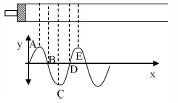Detailed Solution for Part Test - 16 (JEE Advanced) - Question 3

AB

Density of air is maximum at B

Density of air is minimum at D

*Multiple options can be correct
Part Test - 16 (JEE Advanced) - Question 4

A ring of radius R with four metalic wire connected as shown is rolling without sliding on a horizontal surface with a velocity of centre of mass v and angular velocity ω, in a uniform magnetic field B which is perpendicular to the plane of disc as shown in figure. O is the centre of disc and P, Q, R & S are the four points on disc.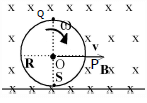Detailed Solution for Part Test - 16 (JEE Advanced) - Question 4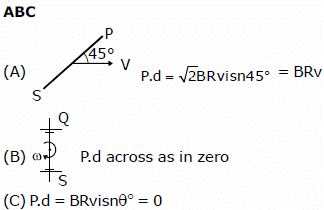Part Test - 16 (JEE Advanced) - Question 5

A prism of a certain angle deviates the red and blue rays by 8°and 12° respectively. Another prism of the same angle deviates the red and blue rays by 10° and 14° respectively. The prisms are small angled and made of different materials. The dispersive powers of the materials of the prisms are in the ratio

Part Test - 16 (JEE Advanced) - Question 6

A radio active substance contains 10,000 nuclei and its half life period is 20 days. The number of nuclei present at the end of 10 days is

Part Test - 16 (JEE Advanced) - Question 7

A ball rests upon a flat piece of paper on a table top. The paper is pulled horizontally but quickly towards right as shown. Relative to its initial position with respect to the table, the ball _____.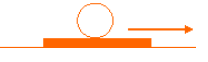(a) remains stationary if there is no friction between the paper and the ball.

(b) moves to the left and starts rolling backwards, i.e. to the left if there is a friction between the paper and the ball.

(c) moves forward, i.e. in the direction in which the paper is pulled.

Here, the correct statement/s is/are _____ .

*Multiple options can be correct
Part Test - 16 (JEE Advanced) - Question 8

A body float on water and also on an oil of density 1.25. Which of the following is/are true ?

Detailed Solution for Part Test - 16 (JEE Advanced) - Question 8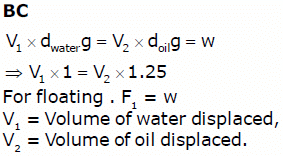Part Test - 16 (JEE Advanced) - Question 9

Two waves are represented by the equations yasin(wkx + 0.57)and y2 = acos(wkx)m, where is in meter and in s. The phase difference between them is

Part Test - 16 (JEE Advanced) - Question 10

A missile is fired for maximum range with an initial velocity of 20 m/s. If g = 10 m /s2, the range of the missile is

Part Test - 16 (JEE Advanced) - Question 11

In the circuit shown, both batteries are ideal. EMF E1 of battery 1 has a fixed value, but emf E2 of battery 2 can be varied between 1 V and 10 V. The graph gives the currents through the two batteries as a function of E2, but are not marked as which plot corresponds to which battery. But for both plots, current is assumed to be negative when the direction of the current through the battery is opposite the direction of that battery's emf. (direction from negative to positive)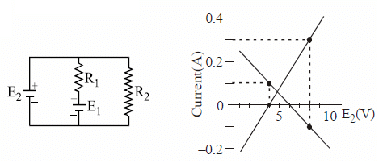Q. The value of emf E1 is

Detailed Solution for Part Test - 16 (JEE Advanced) - Question 11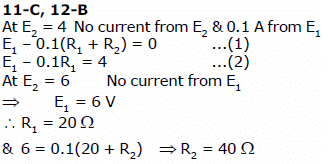Part Test - 16 (JEE Advanced) - Question 12

A and B are two infinitely long straight parallel conductors. C is another straight conductor of length 1 m kept parallel to A and B as shown in the figure. Then the force experienced by C is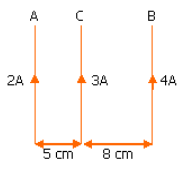Part Test - 16 (JEE Advanced) - Question 13

A mirror is mounted on a stand as shown. The mirror has negligible mass. On the other side, massless arrow is mounted. The natural length of the spring is 1m. The whole system is on frictionless surface. The two bodies are pulled out such that spring is extended by 10 cm and released.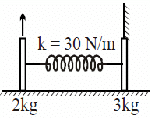Q. What is the minimum distance between arrow and its image during subsequent motion ?

Detailed Solution for Part Test - 16 (JEE Advanced) - Question 13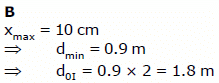Part Test - 16 (JEE Advanced) - Question 14

A mirror is mounted on a stand as shown. The mirror has negligible mass. On the other side, massless arrow is mounted. The natural length of the spring is 1m. The whole system is on frictionless surface. The two bodies are pulled out such that spring is extended by 10 cm and released.Q. What is the maximum velocity of the image of the arrow as seen by a person on the ground ?

Detailed Solution for Part Test - 16 (JEE Advanced) - Question 14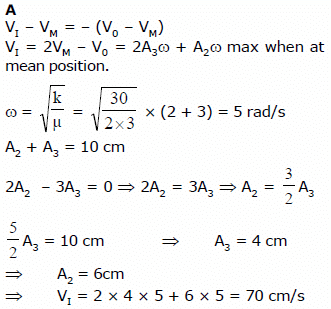Part Test - 16 (JEE Advanced) - Question 15

In the Wheatstone's network given below, P = 10 W, Q = 20W,  R = 15 W, S = 30W The current passing through the battery (of negligible internal resistance) is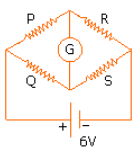Part Test - 16 (JEE Advanced) - Question 16

The current passing through the ideal ammeter in the circuit given below is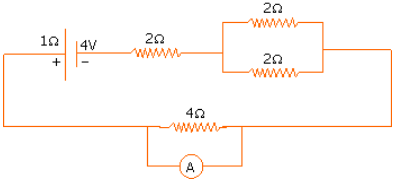Part Test - 16 (JEE Advanced) - Question 17

Arrange the following electromagnetic radiations per quantum in the order of increasing energy:

A : Blue light
B : Yellow light
C : X-ray

Part Test - 16 (JEE Advanced) - Question 18

Out of the following options which one can be used to produce a propagating electromagnetic wave?

Part Test - 16 (JEE Advanced) - Question 19

The electric and magnetic field of an electromagnetic wave are

Part Test - 16 (JEE Advanced) - Question 20

Vapour pressure of pure ‘A’ is 70 mm of Hg at 25 °C. It forms an ideal solution with ‘B’ in which mole fraction of A is 0.8. lf the vapour pressure of the solution is 84 mm of Hg at 25 °C, the vapour pressure of pure ‘B’ at 25 °C is

*Multiple options can be correct
Part Test - 16 (JEE Advanced) - Question 21

Select correct statement(s)?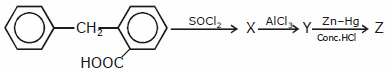Detailed Solution for Part Test - 16 (JEE Advanced) - Question 21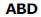are correct answers

*Multiple options can be correct
Part Test - 16 (JEE Advanced) - Question 22

Which of the following increase(s) the activation of a solid adsorbent ?

Detailed Solution for Part Test - 16 (JEE Advanced) - Question 22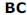are correct amswers

Part Test - 16 (JEE Advanced) - Question 23

When compared to DG0 for the formation of Al2O3 , the AG0 for the formation of Cr2O3 is

*Multiple options can be correct
Part Test - 16 (JEE Advanced) - Question 24

Carbon monooxide is

Detailed Solution for Part Test - 16 (JEE Advanced) - Question 24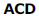are correct answers

*Multiple options can be correct
Part Test - 16 (JEE Advanced) - Question 25

Which of the following is/are endothermic reactions ?

Detailed Solution for Part Test - 16 (JEE Advanced) - Question 25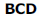are correct answers

*Multiple options can be correct
Part Test - 16 (JEE Advanced) - Question 26

Which of the following are correct order of basic strength ?

Detailed Solution for Part Test - 16 (JEE Advanced) - Question 26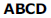are correct answers

*Multiple options can be correct
Part Test - 16 (JEE Advanced) - Question 27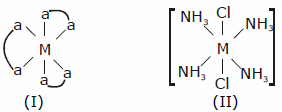Which of the following is correct related to I and II ?

Detailed Solution for Part Test - 16 (JEE Advanced) - Question 27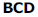are correct answers

Part Test - 16 (JEE Advanced) - Question 28

When conc. H2SO4 is heated with P2 05 , the acid is converted into

Part Test - 16 (JEE Advanced) - Question 29

In Dumas’ method of estimation of nitrogen 0.35 g of an organic compound gave 55 mL of nitrogen collected at 300 K temperature and 715 mm pressure. The percentage composition of nitrogen in the compound would be

(Aqueous tension at 300 K = 15 mm)

Part Test - 16 (JEE Advanced) - Question 30

In which one of the following, does the given amount of chlorine exert the least pressure in a vessel of capacity 1 dm3 at 273K?

Detailed Solution for Part Test - 16 (JEE Advanced) - Question 30

B

Part Test - 16 (JEE Advanced) - Question 31

Enthalpy of vapourization of benzene is +35.3 kJ mol1 at its boiling point of 80°C.

The entropy change in the transition of the vapour to liquid at its boiling point

[in JK1 mol1] is ______.

Part Test - 16 (JEE Advanced) - Question 32

One mole of which of the following has the highest entropy?

Part Test - 16 (JEE Advanced) - Question 33

Number of isomeric forms (constitutional and sterioisomers) for
[Rh(en)2(NO2)(SCN)]+ are

Detailed Solution for Part Test - 16 (JEE Advanced) - Question 33

D

Part Test - 16 (JEE Advanced) - Question 34

One gram of silver gets distributed between 10 cmof molten zinc and 100 cmof molten lead at 800°C. The percentage of silver still left in the lead layer is approximately

Part Test - 16 (JEE Advanced) - Question 35

The activation energy of a reaction at a given temperature is found to be 2.303 RT J mol–1. The ratio of rate constant to the Arrhenius factor is

Part Test - 16 (JEE Advanced) - Question 36

By X-ray studies, the packing of atoms in a crystal of gold is found to be in layers such that starting from any layer, every fourth layer is found to be exactly identical. The density of gold is found to be 19.4 g/cm3 and its atomic mass is 197 amu.

The length of the edge of the unit cell will be:

Detailed Solution for Part Test - 16 (JEE Advanced) - Question 36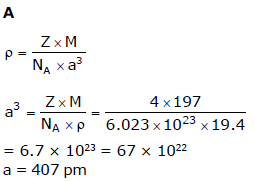Part Test - 16 (JEE Advanced) - Question 37

Which of the following elements is present as the impurity to the maximum extent in the pig iron?

Part Test - 16 (JEE Advanced) - Question 38

Three moles of PCl5, three moles of PCIand two moles of Clz are taken in a closed vessel. If at equilibrium the vessel has 1.5 moles of PCl5, the number of moles of PCl3 present in it is

Part Test - 16 (JEE Advanced) - Question 39

Vapour pressure of pure ‘A’ is 70 mm of Hg at 25 °C. It forms an ideal solution with ‘B’ in which mole fraction of A is 0.8. lf the vapour pressure of the solution is 84 mm of Hg at 25 °C, the vapour pressure of pure ‘B’ at 25 °C is

Part Test - 16 (JEE Advanced) - Question 40

When compared to DG0 for the formation of Al2O3 , the AG0 for the formation of Cr2O3 is

Part Test - 16 (JEE Advanced) - Question 41

When conc. H2SO4 is heated with P2 05 , the acid is converted into

Part Test - 16 (JEE Advanced) - Question 42

The differentiation of sinx with respect to cosx is ?

*Multiple options can be correct
Part Test - 16 (JEE Advanced) - Question 43

Let there are three points A (0, 4/3) B(–1, 0) and C(1, 0) in x – y plane. The distance from a variable point P to the line BC is the geometic mean of the distances from this point to lines AB and AC then locus of P can be

Detailed Solution for Part Test - 16 (JEE Advanced) - Question 43

B, D

Part Test - 16 (JEE Advanced) - Question 44

The number of disulphide linkages present in insulin are

Part Test - 16 (JEE Advanced) - Question 45

The number of gram molecules of chlorine in 6.02 × 1025 hydrogen chloride molecules is

Part Test - 16 (JEE Advanced) - Question 46

One mole of an organic compound ‘A’ with the formula C3H8O reacts completely with two moles of HI to form X and Y. When ‘Y’ is boiled with aqueous alkali forms Z.Z

answers the iodoform test. The compound ‘A’ is ______.

Part Test - 16 (JEE Advanced) - Question 47

One dm3 solution containing 105 moles each of Cl ions and CrO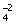ions is treated with 104 mole of silver nitrate. Which one of the following observations is made?

[KSP AgCrO4, = 4 X 1012]

[KSP AgCl = 1 x1010]

Part Test - 16 (JEE Advanced) - Question 48

Decreasing order of reactivity of hydrogen halide acids in the conversion of ROH → RX is

Part Test - 16 (JEE Advanced) - Question 49

The amount of heat evolved when 500 cm3 of 0.1 M HCI is mixed with 200 cm3of 0.2 M NaOH is ______.

Part Test - 16 (JEE Advanced) - Question 50

Standard electrode potential for Sn4+ / Sn2+ couple is +0.15 V and that for the Cr3+ / Cr couple is –0.74 V. These two couples in their standard state are connected to make a cell. The cell potential will be

Detailed Solution for Part Test - 16 (JEE Advanced) - Question 50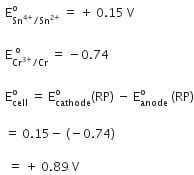Part Test - 16 (JEE Advanced) - Question 51

If one mole of ammonia and one mole of hydrogen chloride are mixed in a closed container to form ammonium chloride gas, then

Part Test - 16 (JEE Advanced) - Question 52

If n = 6, the correct sequence of filling of electrons will be

Part Test - 16 (JEE Advanced) - Question 53

The typical range of molar enthalpies for the strongest intermolecular (Hydrogen) bonds is

Part Test - 16 (JEE Advanced) - Question 54

An organic compound on heating with CuO produces CO2 but no water. The organic compound may be

Part Test - 16 (JEE Advanced) - Question 55

The normality of 30 volume H2O2 is

Detailed Solution for Part Test - 16 (JEE Advanced) - Question 55

B

Part Test - 16 (JEE Advanced) - Question 56

An element with atomic number 21 is a

Part Test - 16 (JEE Advanced) - Question 57

Among the following, the compound that contains ionic, covalent and coordinate linkage is

Part Test - 16 (JEE Advanced) - Question 58

A white crystalline salt A reacts with dilute HCI to liberate a suffocating gas B and also forms a yellow precipitate. The gas B turns potassium dichromate acidified with dilute H2SO4 to a green coloured solution C. A, B and C are respectively ______.

Part Test - 16 (JEE Advanced) - Question 59

For a chemical reaction A → B, the rate of the reaction is 2 × 103 mol dm3 s1, when the initial concentration is 0.05 mol dm3. The rate of the same reaction is 1.6 × 102 mol dm3 s1when the initial concentration is 0.1 mol dm3. The order of the reaction is

Part Test - 16 (JEE Advanced) - Question 60

Excess of carbon dioxide is passed through 50 ml of 0.5 M calcium hydroxide solution.  After the completion of the reaction, the solution was evaporated to dryness. The solid calcium carbonate was completely neutralised with 0.1 N Hydrochloric acid. The volume of Hydrochloric acid required is (At. mass of calcium = 40)

## JEE Main & Advanced Mock Test Series

2 videos|325 docs|189 tests
 Use Code STAYHOME200 and get INR 200 additional OFF Use Coupon Code